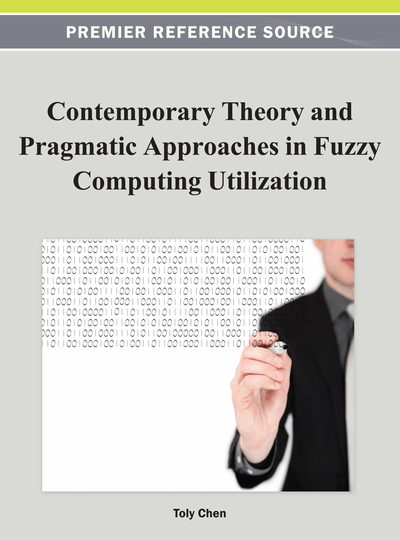# Indirect Adaptive Fuzzy Control for a Class of Uncertain Nonlinear Systems with Unknown Control Direction

Salim Labiod (University of Jijel, Algeria), Hamid Boubertakh (University of Jijel, Algeria) and Thierry Marie Guerra (Université de Valenciennes et du Hainaut-Cambrésis, France)
DOI: 10.4018/978-1-4666-1870-1.ch010

## Abstract

In this paper, the authors propose two indirect adaptive fuzzy control schemes for a class of uncertain continuous-time single-input single-output (SISO) nonlinear dynamic systems with known and unknown control direction. Within these schemes, fuzzy systems are used to approximate unknown nonlinear functions and the Nussbaum gain technique is used to deal with the unknown control direction. This paper first presents a singularity-free indirect adaptive control algorithm for nonlinear systems with known control direction, and then this control algorithm is generalized for the case of unknown control direction. The proposed adaptive controllers are free from singularity, allow initialization to zero of all adjustable parameters of the used fuzzy systems, and guarantee asymptotic convergence of the tracking error to zero. Simulations performed on a nonlinear system are given to show the feasibility of the proposed adaptive control schemes.
Chapter Preview
Top

## Introduction

During the last two decades, there has been significant progress in the area of adaptive control design for nonlinear systems (Slotine & Li, 1991; Krstic et al., 1995; Spooner et al., 2002). Most of the developed adaptive control approaches assume that an accurate model of the system is available and the unknown parameters appear linearly with respect to known nonlinear functions. However, this assumption is not sufficient for many practical situations, because it is difficult to precisely describe a nonlinear system by known nonlinear functions and, therefore, the problem of controlling uncertain nonlinear systems remains a challenging task.

As a model-free design method, fuzzy control has found extensive applications for complex and ill-defined plants (Wang, 1994; Passino & Yurkovich, 1998). In most of these applications, the rule base of the fuzzy controller is constructed from expert knowledge. However, it is sometimes difficult to build the rule base of some plants, or the need may arise to tune the controller parameters if the plant dynamics change. In the hope to overcome this problem, based on the universal approximation theorem and on-line learning ability of fuzzy systems, several stable adaptive fuzzy control schemes have been developed to incorporate the expert knowledge systematically (Wang, 1994). The stability study in such schemes is performed by using the Lyapunov design approach. Conceptually, there are two distinct approaches that have been formulated in the design of a fuzzy adaptive control system: direct and indirect schemes. In the direct scheme, the fuzzy system is used to approximate an unknown ideal controller (Wang, 1994; Spooner & Passino, 1996; Ordonez & Passino, 1999; Chang, 2000; Essounbouli & Hamzaoui, 2006; Labiod & Guerra, 2007). On the other hand, the indirect scheme uses fuzzy systems to estimate the plant dynamics and then synthesizes a control law based on these estimates (Wang, 1994; Ordonez & Passino, 1999; Chang, 2000; Tong et al., 2000; Labiod et al., 2005; Essounbouli & Hamzaoui, 2006; Labiod & Boucherit, 2006). It is worth mentioning that the indirect adaptive approach has the drawback of the controller singularity problem (Labiod et al., 2005; Labiod & Boucherit, 2006).

However, in the aforementioned papers, the control direction is assumed known a priori, i.e., the sign of the control gain is assumed known for the designer. Without this assumption, adaptive controllers design becomes much more difficult, because in this case, one cannot decide the direction along which the control operates and/or the direction of the search of controller parameters. For instance, in indirect fuzzy adaptive control, the control direction is used in the modification of adaptive laws in order to avoid the controller singularity problem and/or in the design of robust control terms to accommodate for approximation errors. In the adaptive control literature, the unknown control direction problem was addressed by (1) using the Nussbaum-type function in the control design for linear and nonlinear systems (Nussbaum, 1983; Ye & Jiang, 1998; Ge et al., 2004; Liu & Huang, 2006; Zhang & Ge, 2007; Liu & Huang, 2008; Boulkroune et al., 2010; Chen & Zhang, 2010) (2) directly estimating unknown parameters involved in the control directions (Kaloust & Qu, 1995; Kaloust & Qu, 1997) (3) the correction vector method for first order nonlinear systems (Brogliato & Lozano, 1994). (4) a switching scheme based on a monitoring function for variable structure model reference adaptive control for linear plants (Lin et al., 2003; Dong et al., 2007) (5) an hysteresis-type function for indirect adaptive fuzzy control (Park et al., 2006; Labiod & Guerra, 2010).

## Complete Chapter List

Search this Book:
Reset